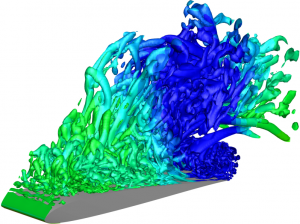# Construction of reduced-order models for fluid flows using deep feedforward neural networks

We present a numerical methodology for construction of reduced-order models (ROMs) of fluid flows through the combination of flow modal decomposition and regression analysis. Spectral proper orthogonal decomposition is applied to reduce the dimensionality of the model and, at the same time, filter the proper orthogonal decomposition temporal modes. The regression step is performed by a deep feedforward neural network (DNN), and the current framework is implemented in a context similar to the sparse identification of nonlinear dynamics algorithm. A discussion on the optimization of the DNN hyperparameters is provided for obtaining the best ROMs and an assessment of these models is presented for a canonical nonlinear oscillator and the compressible flow past a cylinder. Then the method is tested on the reconstruction of a turbulent flow computed by a large eddy simulation of a plunging airfoil under dynamic stall. The reduced-order model is able to capture the dynamics of the leading edge stall vortex and the subsequent trailing edge vortex. For the cases analysed, the numerical framework allows the prediction of the flow field beyond the training window using larger time increments than those employed by the full-order model. We also demonstrate the robustness of the current ROMs constructed via DNNs through a comparison with sparse regression. The DNN approach is able to learn transient features of the flow and presents more accurate and stable long-term predictions compared to sparse regression.Lui, H., & Wolf, W. (2019). Construction of reduced-order models for fluid flows using deep feedforward neural networks. Journal of Fluid Mechanics, 872, 963-994. doi:10.1017/jfm.2019.358